# 3.1 Geometric Modeling

A wide variety of approaches and techniques for geometric modeling exist, and the particular choice usually depends on the application and the difficulty of the problem. In most cases, there are generally two alternatives: 1) a boundary representation, and 2) a solid representation. Suppose we would like to define a model of a planet. Using a boundary representation, we might write the equation of a sphere that roughly coincides with the planet's surface. Using a solid representation, we would describe the set of all points that are contained in the sphere. Both alternatives will be considered in this section.

The first step is to define the world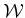for which there are two possible choices: 1) a 2D world, in which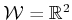, and 2) a 3D world, in which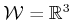. These choices should be sufficient for most problems; however, one might also want to allow more complicated worlds, such as the surface of a sphere or even a higher dimensional space. Such generalities are avoided in this book because their current applications are limited. Unless otherwise stated, the world generally contains two kinds of entities:

1. Obstacles: Portions of the world that are permanently'' occupied, for example, as in the walls of a building.
2. Robots: Bodies that are modeled geometrically and are controllable via a motion plan.
Based on the terminology, one obvious application is to model a robot that moves around in a building; however, many other possibilities exist. For example, the robot could be a flexible molecule, and the obstacles could be a folded protein. As another example, the robot could be a virtual human in a graphical simulation that involves obstacles (imagine the family of Doom-like video games).

This section presents a method for systematically constructing representations of obstacles and robots using a collection of primitives. Both obstacles and robots will be considered as (closed) subsets of. Let the obstacle region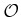denote the set of all points inthat lie in one or more obstacles; hence,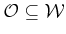. The next step is to define a systematic way of representingthat has great expressive power while being computationally efficient. Robots will be defined in a similar way; however, this will be deferred until Section 3.2, where transformations of geometric bodies are defined.

Subsections
Steven M LaValle 2012-04-20